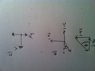# Momentum, Force and Conservation

• Jack Brown
In summary, three smooth stones of masses mA = 8.00 × 10^2 g, mB = 6.00 × 10^2 g, and mC = 2.50 × 10^2 g, initially at rest, are placed in contact with each other on a smooth surface of a frozen lake. An explosion between the stones causes them to fly apart across the surface of the ice, with stone A flying off due north with an initial speed of 2.40 m s^−1 and stone B flying off due east with an initial speed of 3.60 m s^−1. (a) The speed and direction of motion of stone C immediately after the explosion can be calculated

## Homework Statement

Three smooth stones, A, B and C are initially at rest, in contact with each other on the smooth surface of a frozen lake (Figure 1). The masses of the stones are, to 3 significant figures, mA = 8.00 × 10^2 g, mB = 6.00 × 10^2 g and mC =2.50 × 10^2 g and the coefficient of sliding friction between the stones and the ice is μslide = 2.50 × 10^−2. An explosion between the stones causes them to fly apart across the surface of the ice. Stone A flies off due north with an initial speed of 2.40 m s^−1. Stone B flies off due east with an initial speed of 3.60 m s^−1.

(a) Calculate the speed and direction of motion of stone C immediately after the explosion. You can neglect the effects of any exhaust material from the explosion. (Remember that this is a two-dimensional problem and that you should define a suitable coordinate system for your calculation using diagrams where necessary.)

(b) If 10% of the total energy of the explosion was converted to the kinetic
energy of the stones, what was the total energy released in the explosion?

c) Draw a diagram showing all the forces acting on stone A when it is sliding across the ice after the explosion. Calculate how far it travels before it comes to rest. You can assume that the stone does not roll at any time.

## Homework Equations

[/B]
a) momentum = mass x velocity
Pythagoras c^2 = a^2 + b^2
Initial momentum = final momentum

b) Kinetic = 1/2 x mass x velocity^2

c) F=ma where a = μ x g
V = u + at
s = ut + 1/2a(t^2)

## The Attempt at a Solution

[/B]
a)
Momentum A = 0.8 x 2.4 = 1.92
Momentum B = 0.6 x 3.6 = 2.16
Momentum C = 0.25 x V

Using a vector triangle shown in attached picture under a)
initial momentum = final momentum
0 = momentum A + momentum B - momentum C
momentum C = momentum A+ momentum B
From vector triangle
(Mc x Vc)^2 = 1.92^2 + 2.16^2
Vc = (1.92^2)+(2.16^2) / (0.25^2) (m = 0.25)
Vc = 11.6ms-1

Angle Rho = tan^-1 (1.92/2.16) = 41.6 degrees
So the correct direction is is 138.4 degrees below the x axis

b)
Kinetic energy of each disc using 1/2 m v^2
0.5 x 0.8 x 2.4^2 = 2.3
0.5 x 0.6 x 3.6^2 = 3.9
0.5 x 0.25 x 11.6^2 = 16.7

Total energy given in explosion is sum of totals x 10 = 228.6 Joules

c)
Using diagram under b)
Va = 2.4
acceleration = 9.81 x μ
V = u + at
2.4= 0 + 0.25t
t= 2.4/0.25 = 9.6 s

s = ut + 0.5 a t^2
s = 0 + 0.5 x 0.25 x 9.6
s = 11.5m

Please can you help. I struggle with this question.

#### Attachments

•photo.JPG
20.7 KB · Views: 397
Your diagram for a) would be better with labels, A, B, C.
Jack Brown said:
direction is is 138.4 degrees below the x axis
It would be clearer to say 'clockwise from the +ve x axis'. But you are given directions in terms of the compass, so I would answer in those terms.
You diagram for c) seems to show two forces and a velocity. It only asks for forces to be shown. It is a good idea to show the direction of movement, but it would be better to do that with an arrow above the diagram rather than as a line from the stone (which makes it look like a force). If it is meant to be a force it is labelled wrongly. I would also label the vertical upward force.
Jack Brown said:
s = 0 + 0.5 x 0.25 x 9.6
The friction is given as
Jack Brown said:
2.50 × 10^−2

Apart from that, i worked it out right?

Jack Brown said:
Apart from that, i worked it out right?
Yes, looks right, though there is a slightly quicker way. You don't care about time, so use the SUVAT equation that does not involve time. (It's the same as using conservation of energy.)